## First Multiplier Theorem

Let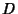be a planar Abelian Difference Set and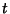be any Divisor of. Thenis a numerical multiplier of, where a multiplier is defined as an automorphismof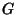which takesto a translation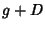of itself for some. Ifis of the form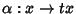for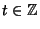relatively prime to the order of, thenis called a numerical multiplier.

References

Gordon, D. M. The Prime Power Conjecture is True for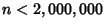.'' Electronic J. Combinatorics 1, R6 1-7, 1994. http://www.combinatorics.org/Volume_1/volume1.html#R6.